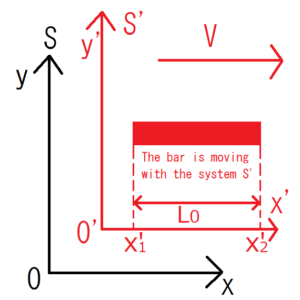Observe moving objects near the light speed from a static system. Then, the object looks shorter than the original length (the length when the object stopped). This phenomenon is called Lorentz contraction.

Let $L_0$ be the length of the bar when the object is stopped and $L$ the length of the moving bar observed from the static system. Then, the following relationship holds between the two. However, it is assumed that the system S’ is moving at a speed V in the x direction with respect to the system S and the bar is moving with the system S’.

\begin{eqnarray} L&=&L_0\sqrt{1-\frac{V^2}{c^2}}\\&=&L_0\sqrt{1-β^2} \end{eqnarray}

## Derivation of Lorentz contraction

### Review of Lorentz transformation

\begin{cases} t’=γ\left(t-\frac{V}{c^2}x \right)\\x’=γ(x-Vt)\\y’=y\\z’=z \end{cases}
$$γ=\frac{1}{\sqrt{1-\frac{V^2}{c^2}}}=\frac{1}{\sqrt{1-β^2}}$$

$$β=\frac{V}{c}$$

### Derivation of Lorentz contractionAs I wrote before, let the system S’ is moving at a speed V in the x direction with respect to the static system S and the bar is moving with the system S’.

The ends of the bar in the system $S$ are denoted by $x_1$ and $x_2$. Likewise, those of the bar in the system $S’$ are denoted by $x’_1$ and $x’_2$. Since the bar is moving with the system $S’$, the bar looks stationary if observers are in the system $S’$. Therefore, the length of the bar $x’_2-x’_1$ observed in the system $S’$ is the same as that of the original bar $L_ 0$.

$$x’_2-x’_1=L_0$$

I’ll show the length of the bar $L$ observed from the static system $S$ using $L_0$.

First, the relationship between $x_1$ and $x’_1$, $x_2$ and $x’_2$ are as follows by Lorentz transformation.

\begin{eqnarray}  \left\{ \begin{array}{l} x’_1=γ(x_1-Vt) \\ x’_2=γ(x_2-Vt) \end{array} \right. \end{eqnarray}

After that substitute the Lorentz transformation above into $L_0=x’_2-x’_1$.

\begin{eqnarray} L_0&=&x’_2-x’_1\\&=&(γ(x_2-Vt))-(γ(x_1-Vt))\\&=&γ(x_2-x_1)\\&=&γL \end{eqnarray}

Divide both sides by $γ$.

$$L=L_0\sqrt{1-\frac{V^2}{c^2}}$$

Considering $\sqrt{1-\frac{V^2}{c^2}}<1$,

$$L_0>L$$

This formula shows the length of the bar $L$ observed from the static system $S$ is shorter than the original length $L_0$.

## Conclusion

Observed from the static system, the moving bar looks shrinking in the direction of travel.